# Sequences & Series Flashcards

Sequences & Series Flashcards
1 / 16 0 0
16 cards in set

## Flashcard Content Overview

This flashcard set will help you study for your next math test or simply brush up on your math knowledge by covering sequences and series. This flashcard set is the perfect study material for arithmetic series, geometric series, and everything in between. Test your knowledge by quizzing yourself with the practice flashcards included, or check your understanding of the formulas for arithmetic and geometric series.

Front
Back
The formula for any arithmetic series is _____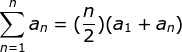Using the arithmetic series, find the sum of the numbers 1 to 25.
The sum of the series is 325. See the setup below: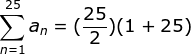The summation notation for 3, 6, 9, 12, 15 looks like _____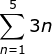Definition of geometric sequence

A pattern of numbers that can be created through repeated multiplication.

Example: The numbers 3, 9, 27, 81 are a geometric sequence. Each number is multiplied by 3 to get the next number in the sequence.

The general rule for the nth term of a geometric series is: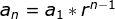Define arithmetic sequence

A sequence that is created through repeated addition.

Example: The numbers 4, 8, 12, 16 are an arithmetic sequence. Each number is added by 4 to get the next number in the sequence.

Identify the next term in this sequence: 10, 19, 28, _____
37 - The next number in this sequence is found by adding 9 to the previous number
The sum of the infinite series 40, 20, 10, 5, ... approaches _____
80 - r = 0.5, so the setup to solve this equation is: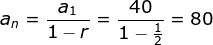Identify the sum of the first five terms in the series that begins at 4 and has a common difference of 6.
80 - The first five terms are 4, 10, 16, 22, and 28. Solving the arithmetic series gives you 80.
The sum of the first five terms in the series 1, 3, 9 ... is _____
121 - The below series solves this problem: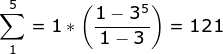The common difference between the terms in the sequence 8, 16, 24, 32, ... is _____
8 - The next term in the sequence is found by adding 8
Evaluate the sum of this series: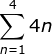The sum of this series is 40.

If we start at n=1, we get 4(1) + 4(2) + 4(3) + 4(4) = 40

Identify the rule in the summation below: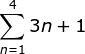The rule is 3n+1
The series below starts at n = _____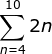n = 4
Evaluate the following: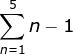The sum of this series is 10.

If we start at n=1, we get (1-1) + (2-1) + (3-1) + (4-1) + (5-1) = 10

Evaluate the sum of the following: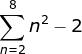The sum of this series is 189.

If we start at n=2, we get (22 - 2) + (32 - 2) + (42 - 2) + (52 - 2) + (62 - 2) + (72 - 2) + (82 - 2) = 189

To unlock this flashcard set you must be a Study.com Member.